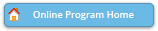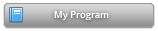Abstract Details

 Activity Number: 254 - Contributed Poster Presentations: Section on Statistical Learning and Data Science Type: Contributed Date/Time: Monday, July 30, 2018 : 2:00 PM to 3:50 PM Sponsor: Section on Statistical Learning and Data Science Abstract #330911 Title: Classification Accuracy of Unsupervised Learning Methods with Discrete and Mixture Distributed Indicators: a Monte Carlo Simulation Study Author(s): Chi Chang* Companies: Keywords: Zero-Inflated; Classification Accuracy; Finite Mixture Model; Model Misspecification; Artificial Neural Network; Cluster Analysis Abstract: This research uses simulations to investigate the classification performance of unsupervised learning methods with discrete data and zero-inflated data. Two mixture components were constructed from 12 indicators, and five different probability distributions were explored: 1) zero-inflated Poisson distribution 2) zero-inflated negative binomial distribution, 3) Poisson distribution, 4) negative binomial distribution, and 5) binomial distribution. For each of the two zero-inflated distributions, proportions of 20% and 70% zeros were studied. Sample sizes of 60, 200, and 3000 were explored for these seven conditions, making a total of 21 scenarios that were explored. Three unsupervised learning methods - cluster analysis (distance measures), latent class analysis (probability approach), and artificial neural network (layer by layer approach) - were utilized to fit the data generated from these scenarios. For each scenario, 500 replications were used. The classification accuracy of each scenario under each method is evaluated, and the potential misuses of unsupervised learning methods are discussed.

Authors who are presenting talks have a * after their name.